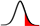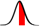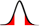# P-value Calculator

Please provide any one value below to compute p-value from z-score or vice versa for a normal distribution.

 Z-score P-value (xZ, right tail)P-value (0 to Z or Z to 0, from center)P-value (-ZZ, two tails)A p-value (probability value) is a value used in statistical hypothesis testing that is intended to determine whether the obtained results are significant. In statistical hypothesis testing, the null hypothesis is a type of hypothesis that states a default position, such as there is no association among groups or relationship between two observations. Assuming that the given null hypothesis is correct, a p-value is the probability of obtaining test results in an experiment that are at least as extreme as the observed results. In other words, determining a p-value helps you determine how likely it is that the observed results actually differ from the null hypothesis.

The smaller the p-value, the higher the significance, and the more evidence there is that the null hypothesis should be rejected for an alternative hypothesis. Typically, a p-value of ≤ 0.05 is accepted as significant and the null hypothesis is rejected, while a p-value > 0.05 indicates that there is not enough evidence against the null hypothesis to reject it.

Given that the data being studied follows a normal distribution, a Z-score table can be used to determine p-values, as in this calculator.